January 20, 2022, 06:13:18 AM

News:

Own IWBasic 2.x ? -----> Get your free upgrade to 3.x now.........

Working with Binary Values

Started by GWS, November 04, 2008, 02:46:47 PM

0 Members and 1 Guest are viewing this topic.

GWS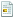November 04, 2008, 02:46:47 PMLast Edit: February 14, 2009, 01:42:49 AM by GWS
Here are a few subroutines doing things with Binary bits .. resurrected from some years ago ..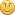You can store 16 status flags in one integer by setting the individual bits to true (=1), or false (=0).

Note that in all of this, the bit positions are numbered right to left, (least significant to most significant), starting at zero ..

Integer value: 538 (Hex value: 0x21A)
Bit Position: 15 14 13 12 11 10 9 8 7 6 5 4 3 2 1 0
Example: 0 0 0 0 0 0 1 0 0 0 0 1 1 0 1 0

Firstly, two subroutines Bin( ) and Dec( ).
Subroutine Bin( ) gives the Binary string corresponding to an integer value, and Subroutine Dec( ) gives the integer value of a Binary string ..

' Two routines (Bin and Dec) to convert Integer to Binary, and back again ..
' GWS Jan 2004 .. re-done 2008
' Creative Basic Code
openconsole
DECLARE Bin(x:int)
DECLARE Dec(s:string)
def d:int
def bstring:string
' Start with Hex value 0xF = 15, which in Binary = "1111" ..
i=0xF
bstring = Bin(i)
print "Binary string corresponding to the integer value 15 .."
print "Binary: ",bstring
print
' convert back again ..
d = Dec(bstring)
print "Convert the Binary string back to an Integer again .."
print "Decimal: ",d
do:until inkey\$<>""
closeconsole
end
sub Bin(x:int)
' converts decimal to binary (allowing for negative values) ..
def j:int
def s:string
j = x
if j < 0 then j = j + 2147483647 + 1
do
s = ltrim\$(str\$(j % 2)) + s
j = j / 2
until j = 0
if x < 0 then s = "1" + s
return s
'endsub
sub Dec(s:string)
' converts binary string to decimal ..
def i,result:int
result = 0
for i = 1 to len(s)
if  mid\$(s,i,1) = "1" then    result = result + 2 ^ (len(s) - i)
next i

return result

Next, if you are going to work with status flags, you need a means of setting a given bit to '1' if a condition is True, a way of testing a given bit to see if it's set or not, and finally a way of clearing (turning off) each bit if the condition it represents is False ..
Here are three subroutines Set( ), Test( ), and Off( ) which do these things ..

' Three routines (Set, Test, and Off) to turn on, test, and turn off bits ..
' GWS Jan 2004 .. re-done 2008
' Creative Basic Code
openconsole
DECLARE Bin(x:int)
DECLARE Set(x:int,bit:int)
DECLARE Test(x:int,bit:int)
DECLARE Off(x:int,bit:int)
def v,i,b:int
def bstring:string
i = 8 :' initial value is representing '1000' which is 8 in decimal ..
b = 2 :' we want to set bit 2 ..
' Note bits are numbered right to left starting at zero, so this will set the third
' bit from the right ..
' Set the bit ..
v = Set(i,b)
' update the integer value representing the binary string ...
i = v
' get the new binary string ..
bstring = Bin(v)
print "Check that Bit 2, the third bit from the right has been set .."
print "Bit string: " + bstring
print "Stored as integer: " + str\$(i)
print
' now Test if bit 2 is set ..
' it should be 'cos we just set it ..
v = Test(v,b)
print "Check whether Bit 2 is set .."
if v = 1
print "Yes, Bit ",b," is set."
else
print "No, Bit ",b," is not set."
endif
print
' now we Clear the fourth bit from the right ..
b = 3
' Clear the fourth bit from the right ..
v = Off(i,b)
' get the new binary string ..
bstring = Bin(v)
print "Check that Bit 3 (the fourth from the right), has been cleared .."
print "Bit string: " + bstring
print "Stored as integer: " + str\$(v)
do:until inkey\$<>""
closeconsole
end
sub Set(x:int,bit:int)
def j:int
' sets bit 'b' in an integer representing a binary string ..
j = i | (2 ^ bit)
return j
'endsub
sub Test(x:int,bit:int)
def j:int
' tests whether bit 'b' is set, in an integer representing a binary string ..
j = x / (2 ^ bit) % 2
return j
'endsub
sub Off(x:int,bit:int)
def j:int
' Clears bit 'bit' in an integer representing a binary string ..
j = x & ((2 ^ bit) || 0xFFFFFFFF)
return j
'endsub
sub Bin(x:int)
' converts decimal to binary (allowing for negative values) ..
def j:int
def s:string
j = x
if j < 0 then j = j + 2147483647 + 1
do
s = ltrim\$(str\$(j % 2)) + s
j = j / 2
until j = 0
if x < 0 then s = "1" + s
return s

[Edit Feb 2009 .. see Egil's post lower down.   Amended sub Bin() for error in the case of negative numbers.]

all the best,Graham
Tomorrow may be too late ..

aurelCBNovember 05, 2008, 10:37:33 AM #1
So this sign is %
Modulus.  The remainder of an integer division
In some other basic is written like MOD.(VB)
Good example Graham!forum for hobby programming:<br />https://aurelsoft.ucoz.com

GWSNovember 05, 2008, 12:17:04 PM #2
Yep ..

n = 5 % 3

gives a result remainder n= 2

All sorts of amazing stuff in Creative ..best wishes,

Graham
Tomorrow may be too late ..

EgilFebruary 13, 2009, 08:56:29 AM #3
Graham:
I have been studying these routines very closely, but there is one thing I don't understand.
In the Bin subroutine you have a line reading    if i < 0 then j = j + 2147483647 + 1.
How did you figure out the the value 2147483647??
Support Amateur Radio  -  Have a ham  for dinner!

REDEBOLTFebruary 13, 2009, 09:49:29 AM #4
2147483647  = 2^31 - 1
Regards,
Bob

EgilFebruary 13, 2009, 01:32:02 PM #5
Thanks a lot Bob.
I should have seen that myself. But as we say here in Norway: Sometimes you can't see the wood for all the trees.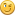Support Amateur Radio  -  Have a ham  for dinner!

GWSFebruary 13, 2009, 01:39:19 PM #6
Hi Egil,

As Bob says 2147483647 = 2^31 - 1

It's to do with two's-complement storage where -1 = 0xFFFFFFFFFFFFFFFF
The leading bit is '0' for a positive number, and '1' for negative numbers.

It's been ages since I looked at that.  It was a lot of bits of code I gathered from the Net and converted to Basic.

Actually, if anyone wanted to set individual bits as flags, it would make more sense to define the variable as UINT, so negative values wouldn't be used anyway.

Looking at the test program again though, I think there were a couple of errors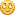I think this is the right version:

sub Bin(x:int)
' converts decimal to binary (allowing for negative values) ..
def j:int
def s:string
j = x
if (j < 0) then j = j + 2147483647 + 1

do
s = ltrim\$(str\$(j % 2)) + s
j = j / 2
until j = 0
if (x < 0) then s = "1" + s
return s

The previous version was missing a '1' for negative values ..

I shall have to re-visit this topic .. but it will take a bit of concentrated thought- or in other words, it might take me a while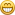best wishes,Graham
Tomorrow may be too late ..

Egil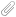February 14, 2009, 08:19:10 AM #7 Last Edit: February 14, 2009, 11:04:29 AM by Egil
Hi Graham,
Lately I have been playing around with an AIS decoding receiver. The receiver output delivers messages to a RS232-C line, where the information consists of  28 characters, each representing a  6 bit "message". These "messages" adds up to a 168 bit sentence containing a vessels navigational status. The different parts of information all have different numbers of bits, ranging from 1 to 28 bits. The longest ones represents the vessels position in "signed binaries".

There are several software packages on the market that can present the decoded data on a map, but they all lack proper routines for communicating with other computers, and for saving a database that can be accessed later, either from the same software, or from other software. So I have decided to "roll my own".

Last weekend I started to work with this particular problem, and some of the needed decoding routines was made. Therefore i was delighted to see that your Dec subroutine was almost identical to what I have come up with. I enclose my routine for converting the 28 bit longitude value to decimal. It is coded with EB, but the code will be almost identical with CB.

73's de LA2PJ

Support Amateur Radio  -  Have a ham  for dinner!